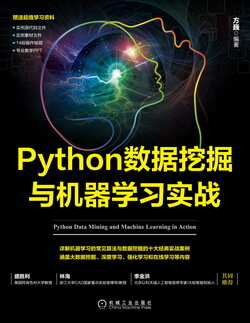# Python 数据挖掘与机器学习实战 (72)：回归分析介 3.6.3(梯度下降法求解最小值)

1．_θ_ 更新过程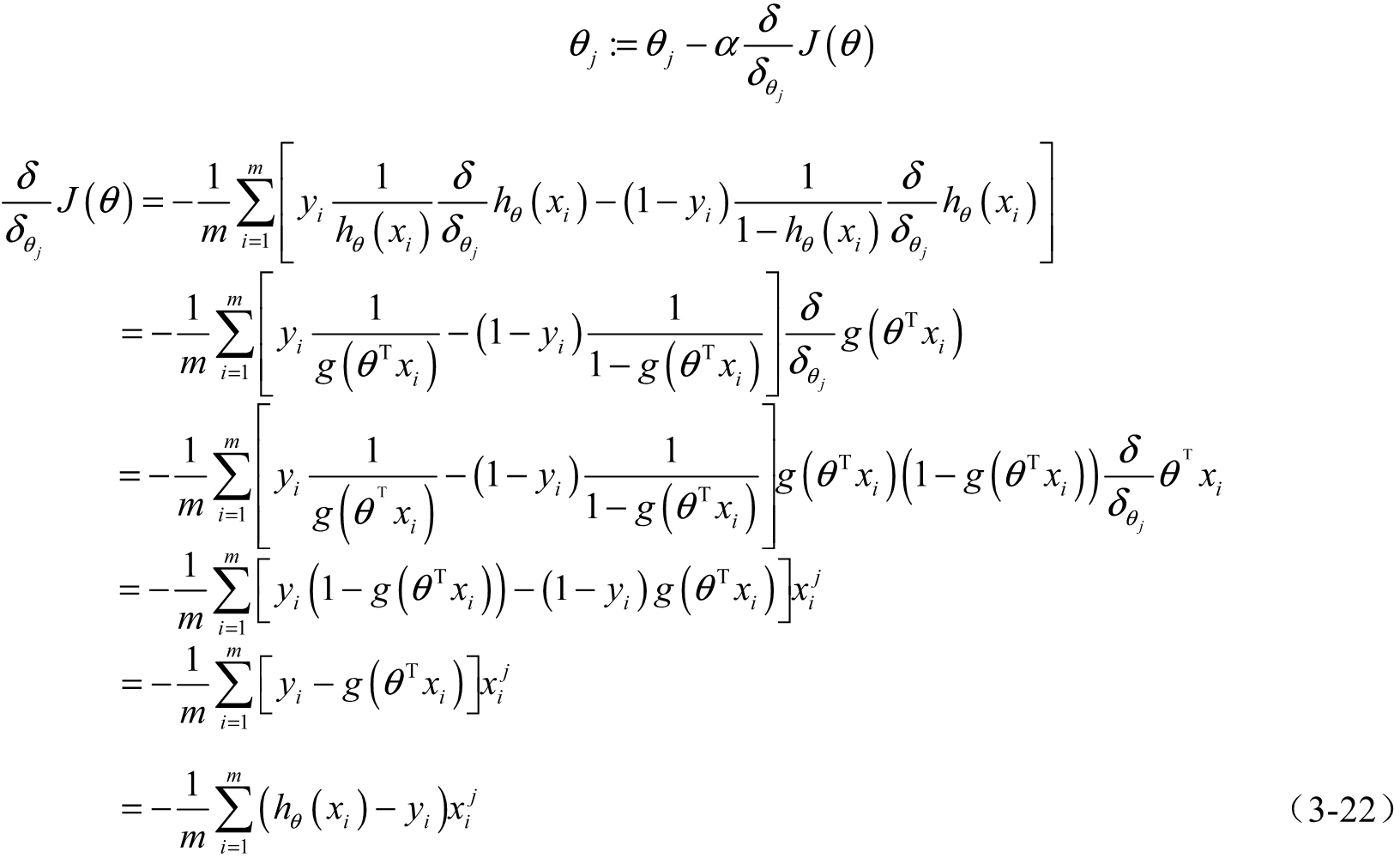$\theta$$\theta$ 更新过程可以写成：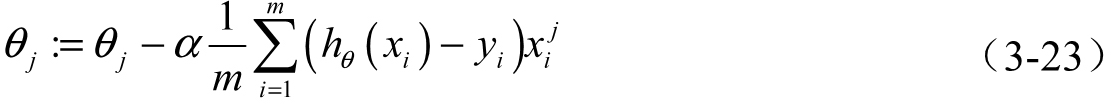2．向量化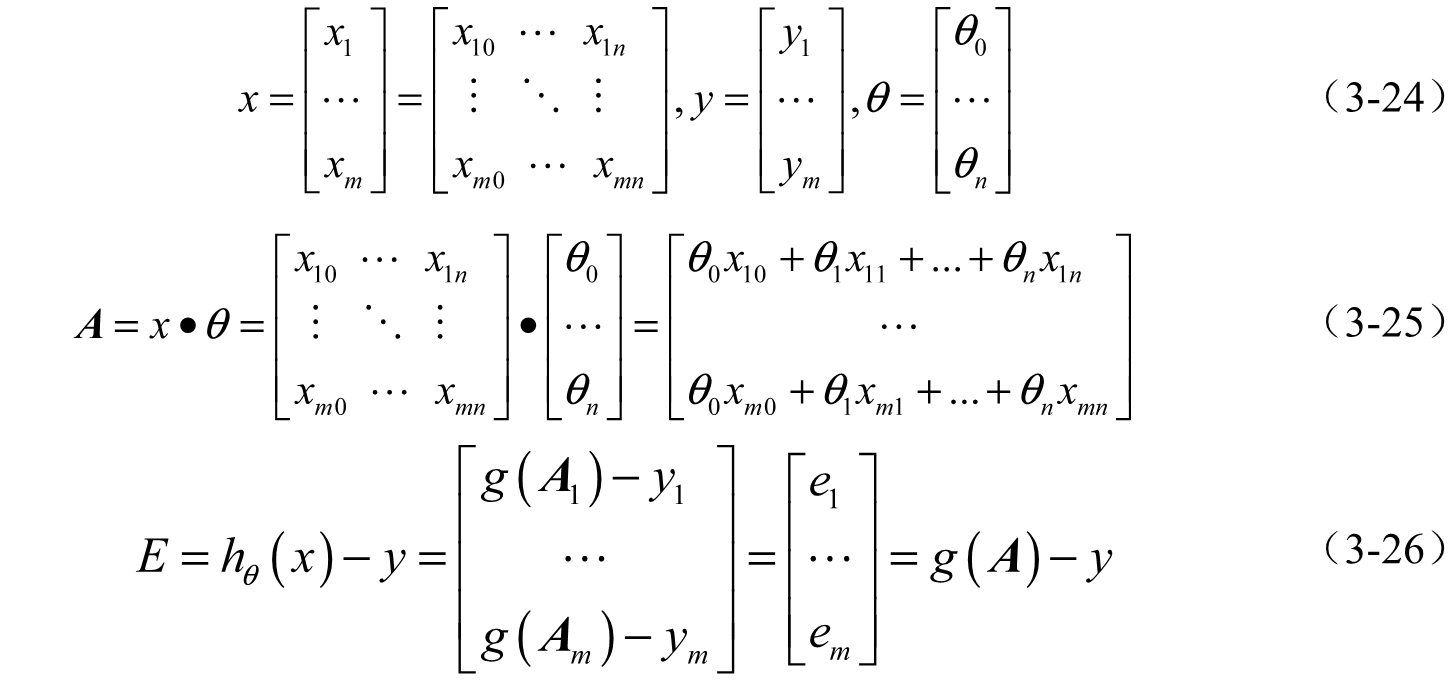$g\left(A\right)$$g(A)$ 的参数 $A$$A$ 为一列向量，所以实现 $g$$g$ 函数时要支持列向量作为参数，并返回列向量。$\theta$$\theta$ 更新过程可以改为：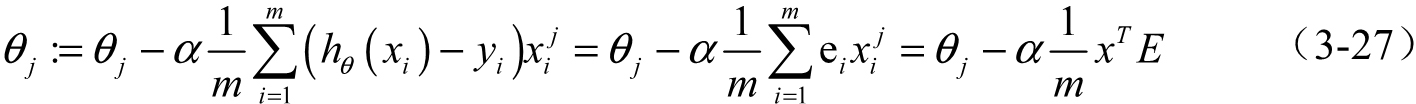3．正则化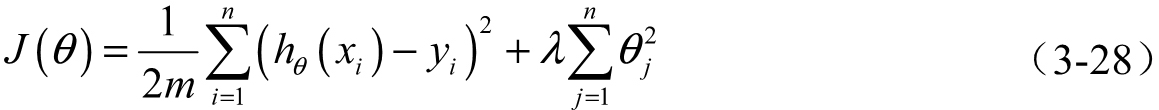$\lambda$$\lambda$ 是正则项系数：

• 如果它的值很大，说明对模型的复杂度惩罚大，对拟合数据的损失惩罚小，这样它就不会过分拟合数据，在训练数据上的偏差较大，在未知数据上的方差较小，但是可能出现欠拟合的现象。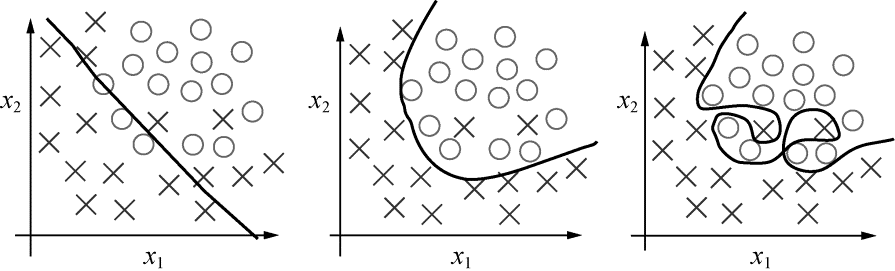• 如果它的值很小，说明比较注重对训练数据的拟合，在训练数据上的偏差会小，但是可能会导致过拟合。

${\theta }_{j}:={\theta }_{j}-\frac{\alpha }{m}\sum _{i=1}^{m}\left({h}_{\theta }\left({x}_{i}\right)-{y}_{i}\right){x}_{i}^{j}-\frac{\lambda }{m}{\theta }_{j}\phantom{\rule{1em}{0ex}}\phantom{\rule{1em}{0ex}}\phantom{\rule{1em}{0ex}}\phantom{\rule{1em}{0ex}}\phantom{\rule{1em}{0ex}}\phantom{\rule{1em}{0ex}}\phantom{\rule{1em}{0ex}}\left(3-29\right)$$\theta_j:=\theta_j-\frac{\alpha}{m}\sum\limits^m_{i=1}(h_{\theta}(x_i)-y_i)x^j_i-\frac{\lambda}{m}\theta_j\quad\quad\quad\quad\quad\quad\quad(3-29)$